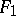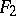# Erlangen program

(diff) ← Older revision | Latest revision (diff) | Newer revision → (diff)
The essence of the Erlangen program consists in the following. As is well known, Euclidean geometry considers the properties of figures that do not change under rigid motions; equal figures are defined as those that can be transferred onto one another by a motion. But instead of motions one may choose any other collection of geometric transformations and declare as "equal" those figures that are obtained from one another by transformations of this collection. This leads to another "geometry" , which studies the properties of figures that do not change under such transformations. The relevant "equality" must satisfy the following three natural conditions: 1) every figureis "equal" to itself; 2) if a figureis "equal" to, thenis "equal" to; and 3) ifis "equal" toandto, thenis "equal" to. Accordingly, one has to require that the collection of transformations in question is a group. The theory that studies the properties of figures that are preserved under all transformations of a given group is called the geometry of this group.